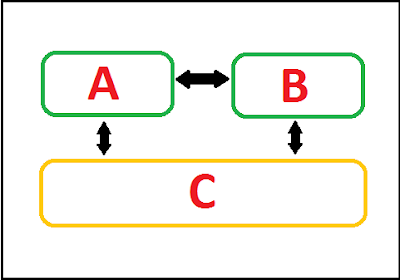# Zeroth Law Of ThermodynamicsZeroth law of thermodynamics mainly deals with one of the most important properties of thermodynamic system i.e. temperature.

Temperature is a property which denotes the degree of hotness or coldness of a system or any matter.

Here we are talking about temperature so it is clear that zeroth law of thermodynamics is a law of temperature.

### The principle of Temperature Measurement

After the recognition of temperature as a property, the measurement of this property is required. Temperature is not an actual parameter, that is why temperature cannot be measured as its actual value so there are some standards made to define and measure this property.

Some standard temperature are used as consideration to develop these units, such as ice point ( temperature of ice considered to be 0°C, Steam point (temperature of water when steam formation starts is considered to be 100°C)

There are some more fixed temperatures like this referring to which the temperature scales were developed.

### Zeroth law of thermodynamics Statement

Zeroth law of thermodynamics states." When two bodies A and B are separately in thermal equilibrium with another body C, then these two bodies A and B will also be in thermal equilibrium."Zeroth law of thermodynamics
The block diagram above shows that the Body A is in equilibrium with body C and body B is also in equilibrium with body C. this will make both A and B in equilibrium. Equilibrium is shown by arrows in the block diagram.

Zeroth law pays a vital role in the temperature measurement and analysis of thermal equilibrium. Various types of Thermometer is developed with the principle of temperature measurement.

Zeroth law of thermodynamics was discovered after the first and second law of thermodynamics. But the law should be placed before the first and second law, that is why it was named Zeroth law of thermodynamics.

#### 1 comment:

1.